# Neural Network In Python: Introduction, Structure And Trading Strategies

You are probably wondering how a technical topic like Neural Network Tutorial is hosted on an algorithmic trading website. Neural network studies were started in an effort to map the human brain and understand how humans take decisions but algorithm tries to remove human emotions altogether from the trading aspect. What we sometimes fail to realise is that the human brain is quite possibly the most complex machine in this world and has been known to be quite effective at coming to conclusions in record time.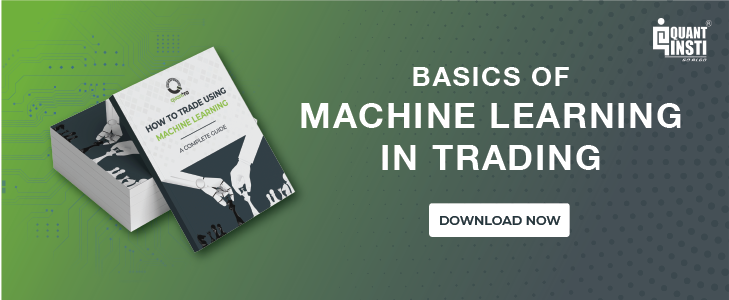Think about it, if we could harness the way our brain works and apply it in the machine learning domain (Neural networks are after all a subset of machine learning), we could possibly take a giant leap in terms of processing power and computing resources.

Before we dive deep into the nitty-gritty of neural network trading, we should understand the working of the principal component, ie the neuron. Thus, in the neural network tutorial, we will cover the following topics

Remember, the end goal of the neural network tutorial is to understand the concepts involved in neural networks and how they can be applied to predict stock prices in the live markets. Let us start by understanding what a neuron is.

## Structure of a Neuron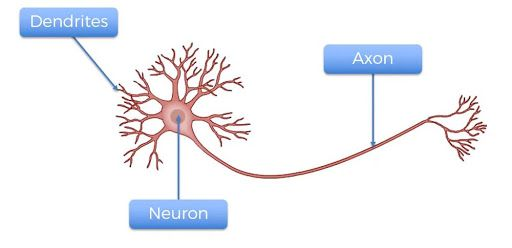There are three components to a neuron, the dendrites, axon and the main body of the neuron. The dendrites are the receivers of the signal and the axon is the transmitter. Alone, a neuron is not of much use, but when it is connected to other neurons, it does several complicated computations and helps operate the most complicated machine on our planet, the human body.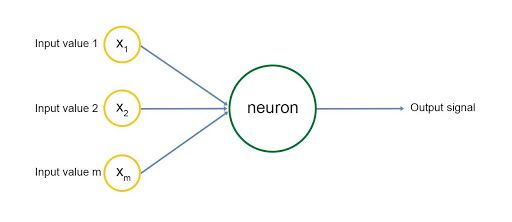## Perceptron: the Computer Neuron

A perceptron ie a computer neuron is built in a similar manner, as shown in the diagram.

There are inputs to the neuron marked with yellow circles, and the neuron emits an output signal after some computation.

The input layer resembles the dendrites of the neuron and the output signal is the axon. Each input signal is assigned a weight, wi. This weight is multiplied by the input value and the neuron stores the weighted sum of all the input variables. These weights are computed in the training phase of the neural network learning through concepts called gradient descent and backpropagation, we will cover these topics later on.

An activation function is then applied to the weighted sum, which results in the output signal of the neuron.

The input signals are generated by other neurons, i.e, the output of other neurons, and the network is built to make predictions/computations in this manner.

This is the basic idea of a neural network. We will look at each of these concepts in more detail in this neural network tutorial.

## Understanding a Neural Network

We will look at an example to understand the working of neural networks. The input layer consists of the parameters that will help us arrive at an output value or make a prediction. Our brains essentially have five basic input parameters, which are our senses to touch, hear, see, smell and taste.

The neurons in our brain create more complicated parameters such as emotions and feelings, from these basic input parameters. And our emotions and feelings, make us act or take decisions which is basically the output of the neural network of our brains. Therefore, there are two layers of computations in this case before making a decision.

The first layer takes in the five senses as inputs and results in emotions and feelings, which are the inputs to the next layer of computations, where the output is a decision or an action.

Hence, in this extremely simplistic model of the working of the human brain, we have one input layer, two hidden layers, and one output layer. Of course from our experiences, we all know that the brain is much more complicated than this, but essentially this is how the computations are done in our brain.

## Neural Network In Trading: An Example

To understand the working of a neural network in trading, let us consider a simple stock price prediction example, where the OHLCV (Open-High-Low-Close-Volume) values are the input parameters, there is one hidden layer and the output consists of the prediction of the stock price.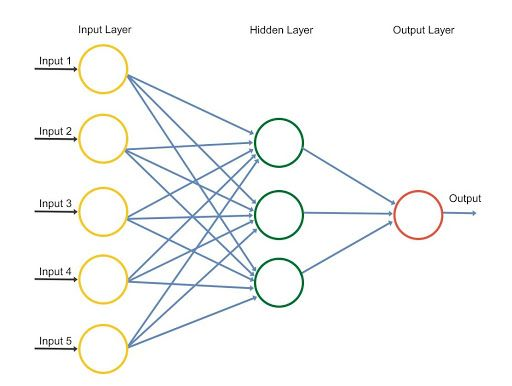In the example taken in the neural network tutorial, there are five input parameters as shown in the diagram.

The hidden layer consists of 3 neurons and the resultant in the output layer is the prediction for the stock price.

The 3 neurons in the hidden layer will have different weights for each of the five input parameters and might have different activation functions, which will activate the input parameters according to various combinations of the inputs.

For example, the first neuron might be looking at the volume and the difference between the Close and the Open price and might be ignoring the High and Low prices. In this case, the weights for High and Low prices will be zero.

Based on the weights that the model has trained itself to attain, an activation function will be applied to the weighted sum in the neuron, this will result in an output value for that particular neuron.

Similarly, the other two neurons will result in an output value based on their individual activation functions and weights. Finally, the output value or the predicted value of the stock price will be the sum of the three output values of each neuron. This is how the neural network will work to predict stock prices.

Now that you understand the working of a neural network, we will move to the heart of the matter of this neural network tutorial, and that is learning how the Artificial Neural Network will train itself to predict the movement of a stock price.

## Training the Neural Network

To simplify things in the neural network tutorial, we can say that there are two ways to code a program for performing a specific task.

• Define all the rules required by the program to compute the result given some input to the program.
• Develop the framework upon which the code will learn to perform the specific task by training itself on a dataset through adjusting the result it computes to be as close to the actual results which have been observed.

The second process is called training the model which is what we will be focussing on. Let’s look at how our neural network will train itself to predict stock prices.

The neural network will be given the dataset, which consists of the OHLCV data as the input, as well as the output, we would also give the model the Close price of the next day, this is the value that we want our model to learn to predict. The actual value of the output will be represented by ‘y’ and the predicted value will be represented by y^, y hat.

The training of the model involves adjusting the weights of the variables for all the different neurons present in the neural network. This is done by minimizing the ‘Cost Function’. The cost function, as the name suggests is the cost of making a prediction using the neural network. It is a measure of how far off the predicted value, y^, is from the actual or observed value, y.

There are many cost functions that are used in practice, the most popular one is computed as half of the sum of squared differences between the actual and predicted values for the training dataset.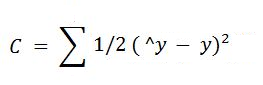The way the neural network trains itself is by first computing the cost function for the training dataset for a given set of weights for the neurons. Then it goes back and adjusts the weights, followed by computing the cost function for the training dataset based on the new weights. The process of sending the errors back to the network for adjusting the weights is called backpropagation.

This is repeated several times till the cost function has been minimized. We will look at how the weights are adjusted and the cost function is minimized in more detail next.

The weights are adjusted to minimize the cost function. One way to do this is through brute force. Suppose we take 1000 values for the weights, and evaluate the cost function for these values. When we plot the graph of the cost function, we will arrive at a graph as shown below.

The best value for weights would be the cost function corresponding to the minima of this graph.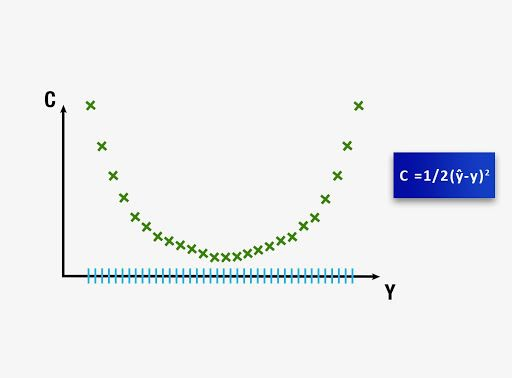This approach could be successful for a neural network involving a single weight which needs to be optimized. However, as the number of weights to be adjusted and the number of hidden layers increases, the number of computations required will increase drastically.

The time it will require to train such a model will be extremely large even on the world’s fastest supercomputer. For this reason, it is essential to develop a better, faster methodology for computing the weights of the neural network. This process is called Gradient Descent. We will look into this concept in the next part of the neural network tutorial.

Gradient descent involves analyzing the slope of the curve of the cost function. Based on the slope we adjust the weights, to minimize the cost function in steps rather than computing the values for all possible combinations.

The visualization of Gradient descent is shown in the diagrams below. The first plot is a single value of weights and hence is two dimensional. It can be seen that the red ball moves in a zig-zag pattern to arrive at the minimum of the cost function.

In the second diagram, we have to adjust two weights in order to minimize the cost function. Therefore, we can visualize it as a contour, as shown in the graph, where we are moving in the direction of the steepest slope, in order to reach the minima in the shortest duration. With this approach, we do not have to do many computations and as a result, the computations do not take very long, making the training of the model a feasible task.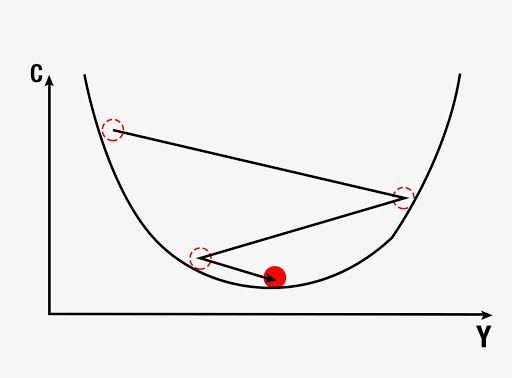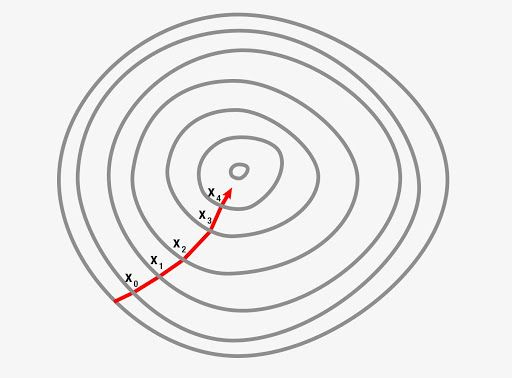Gradient descent can be done in three possible ways,

In batch gradient descent, the cost function is computed by summing all the individual cost functions in the training dataset and then computing the slope and adjusting the weights.

In stochastic gradient descent, the slope of the cost function and the adjustments of weights are done after each data entry in the training dataset. This is extremely useful to avoid getting stuck at a local minima if the curve of the cost function is not strictly convex. Each time you run the stochastic gradient descent, the process to arrive at the global minima will be different. Batch gradient descent may result in getting stuck with a suboptimal result if it stops at local minima.

The third type is the mini-batch gradient descent, which is a combination of the batch and stochastic methods. Here, we create different batches by clubbing together multiple data entries in one batch. This essentially results in implementing the stochastic gradient descent on bigger batches of data entries in the training dataset.

While we can dive deep into Gradient descent, we are afraid it will be outside the scope of the neural network tutorial. Hence let’s move forward and understand how backpropagation works to adjust the weights according to the error which had been generated.

## Backpropagation

Backpropagation is an advanced algorithm which enables us to update all the weights in the neural network simultaneously. This drastically reduces the complexity of the process to adjust weights. If we were not using this algorithm, we would have to adjust each weight individually by figuring out what impact that particular weight has on the error in the prediction. Let us look at the steps involved in training the neural network with Stochastic Gradient Descent:

• Initialize the weights to small numbers very close to 0 (but not 0)
• Forward propagation - the neurons are activated from left to right, by using the first data entry in our training dataset, until we arrive at the predicted result y
• Measure the error which will be generated
• Backpropagation - the error generated will be backpropagated from right to left, and the weights will be adjusted according to the learning rate
• Repeat the previous three steps, forward propagation, error computation and backpropagation on the entire training dataset
• This would mark the end of the first epoch, the successive epochs will begin with the weight values of the previous epochs, we can stop this process when the cost function converges within a certain acceptable limit

We have covered a lot in this neural network tutorial and this leads us to apply these concepts in practice. Thus, we will now learn how to develop our own Artificial Neural Network to predict the movement of a stock price.

You will understand how to code a strategy using the predictions from a neural network that we will build from scratch. You will also learn how to code the Artificial Neural Network in Python, making use of powerful libraries for building a robust trading model using the power of Neural Networks.

## Coding the Strategy

### Importing Libraries

We will start by importing a few libraries, the others will be imported as and when they are used in the program at different stages. For now, we will import the libraries which will help us in importing and preparing the dataset for training and testing the model.

import numpy as np
import pandas as pd
import talib

Numpy is a fundamental package for scientific computing, we will be using this library for computations on our dataset. The library is imported using the alias np.

Pandas will help us in using the powerful dataframe object, which will be used throughout the code for building the artificial neural network in Python.

Talib is a technical analysis library, which will be used to compute the RSI and Williams %R. These will be used as features for training our artificial neural network. We could add more features using this library.

### Setting the random seed to a fixed number

import random
random.seed(42)

Random will be used to initialize the seed to a fixed number so that every time we run the code we start with the same seed.

### Importing the dataset

dataset = pd.read_csv('RELIANCE.NS.csv')
dataset = dataset.dropna()
dataset = dataset[['Open', 'High', 'Low', 'Close']]

We then import our dataset, which is stored in the .csv file named ‘RELIANCE.NS.csv’. This is done using the pandas library, and the data is stored in a dataframe named dataset. We then drop the missing values in the dataset using the dropna() function. The csv file contains daily OHLC data for the stock of Reliance trading on NSE for the time period from 1st January 1996 to 15th January 2018.

We choose only the OHLC data from this dataset, which would also contain the date, Adjusted Close and Volume data. We will be building our input features by using only the OHLC values.

### Preparing the dataset

dataset['H-L'] = dataset['High'] - dataset['Low']
dataset['O-C'] = dataset['Close'] - dataset['Open']
dataset['3day MA'] = dataset['Close'].shift(1).rolling(window = 3).mean()
dataset['10day MA'] = dataset['Close'].shift(1).rolling(window = 10).mean()
dataset['30day MA'] = dataset['Close'].shift(1).rolling(window = 30).mean()
dataset['Std_dev']= dataset['Close'].rolling(5).std()
dataset['RSI'] = talib.RSI(dataset['Close'].values, timeperiod = 9)
dataset['Williams %R'] = talib.WILLR(dataset['High'].values, dataset['Low'].values, dataset['Close'].values, 7)

We then prepare the various input features which will be used by the artificial neural network learning for making the predictions. We define the following input features:

• High minus Low price
• Close minus Open price
• Three day moving average
• Ten day moving average
• 30 day moving average
• Standard deviation for a period of 5 days
• Relative Strength Index
• Williams %R

dataset['Price_Rise'] = np.where(dataset['Close'].shift(-1) > dataset['Close'], 1, 0)

We then define the output value as price rise, which is a binary variable storing 1 when the closing price of tomorrow is greater than the closing price of today.

dataset = dataset.dropna()

Next, we drop all the rows storing NaN values by using the dropna() function.

X = dataset.iloc[:, 4:-1]
y = dataset.iloc[:, -1]

We then create two data frames storing the input and the output variables. The dataframe ‘X’ stores the input features, the columns starting from the fifth column (or index 4) of the dataset till the second last column. The last column will be stored in the dataframe y, which is the value we want to predict, i.e. the price rise.

### Splitting the dataset

split = int(len(dataset)*0.8)
X_train, X_test, y_train, y_test = X[:split], X[split:], y[:split], y[split:]

In this part of the code, we will split our input and output variables to create the test and train datasets. This is done by creating a variable called split, which is defined to be the integer value of 0.8 times the length of the dataset.

We then slice the X and y variables into four separate data frames: Xtrain, Xtest, ytrain and ytest. This is an essential part of any machine learning algorithm, the training data is used by the model to arrive at the weights of the model. The test dataset is used to see how the model will perform on new data which would be fed into the model. The test dataset also has the actual value for the output, which helps us in understanding how efficient the model is. We will look at the confusion matrix later in the code, which essentially is a measure of how accurate the predictions made by the model are.

### Feature Scaling

from sklearn.preprocessing import StandardScaler
sc = StandardScaler()
X_train = sc.fit_transform(X_train)
X_test = sc.transform(X_test)

Another important step in data preprocessing is to standardize the dataset. This process makes the mean of all the input features equal to zero and also converts their variance to 1. This ensures that there is no bias while training the model due to the different scales of all input features. If this is not done the neural network might get confused and give a higher weight to those features which have a higher average value than others.

We implement this step by importing the StandardScaler method from the sklearn.preprocessing library. We instantiate the variable sc with the StandardScaler() function. After which we use the fittransform function for implementing these changes on the Xtrain and Xtest datasets. The ytrain and y_test sets contain binary values, hence they need not be standardized. Now that the datasets are ready, we may proceed with building the Artificial Neural Network using the Keras library.

### Building the Artificial Neural Network

from keras.models import Sequential
from keras.layers import Dense
from keras.layers import Dropout

Now we will import the functions which will be used to build the artificial neural network. We import the Sequential method from the keras.models library. This will be used to sequentially build the layers of the neural networks learning. The next method that we import will be the Dense function from the keras.layers library.

This method will be used to build the layers of our artificial neural network.

classifier = Sequential()

We instantiate the Sequential() function into the variable classifier. This variable will then be used to build the layers of the artificial neural network learning in python.

classifier.add(Dense(units = 128, kernel_initializer = 'uniform', activation = 'relu', input_dim = X.shape))

To add layers into our Classifier, we make use of the add() function. The argument of the add function is the Dense() function, which in turn has the following arguments:

• Units: This defines the number of nodes or neurons in that particular layer. We have set this value to 128, meaning there will be 128 neurons in our hidden layer.
• Kernel_initializer: This defines the starting values for the weights of the different neurons in the hidden layer. We have defined this to be ‘uniform’, which means that the weights will be initialized with values from a uniform distribution.
• Activation: This is the activation function for the neurons in the particular hidden layer. Here we define the function as the rectified Linear Unit function or ‘relu’.
• Input_dim: This defines the number of inputs to the hidden layer, we have defined this value to be equal to the number of columns of our input feature dataframe. This argument will not be required in the subsequent layers, as the model will know how many outputs the previous layer produced.
classifier.add(Dense(units = 128, kernel_initializer = 'uniform', activation = 'relu'))

We then add a second layer, with 128 neurons, with a uniform kernel initializer and ‘relu’ as its activation function. We are only building two hidden layers in this neural network.

classifier.add(Dense(units = 1, kernel_initializer = 'uniform', activation = 'sigmoid'))

The next layer that we build will be the output layer, from which we require a single output. Therefore, the units passed are 1, and the activation function is chosen to be the Sigmoid function because we would want the prediction to be a probability of market moving upwards.

classifier.compile(optimizer = 'adam', loss = 'mean_squared_error', metrics = ['accuracy'])

Finally, we compile the classifier by passing the following arguments:

• Optimizer: The optimizer is chosen to be ‘adam’, which is an extension of the stochastic gradient descent.
• Loss: This defines the loss to be optimized during the training period. We define this loss to be the mean squared error.
• Metrics: This defines the list of metrics to be evaluated by the model during the testing and training phase. We have chosen accuracy as our evaluation metric.
classifier.fit(X_train, y_train, batch_size = 10, epochs = 100)

Now we need to fit the neural network that we have created to our train datasets. This is done by passing Xtrain, ytrain, batch size and the number of epochs in the fit() function. The batch size refers to the number of data points that the model uses to compute the error before backpropagating the errors and making modifications to the weights. The number of epochs represents the number of times the training of the model will be performed on the train dataset.

With this, our artificial neural network in Python has been compiled and is ready to make predictions.

### Predicting the movement of the stock

y_pred = classifier.predict(X_test)
y_pred = (y_pred > 0.5)

Now that the neural network has been compiled, we can use the predict() method for making the prediction. We pass Xtest as its argument and store the result in a variable named ypred. We then convert ypred to store binary values by storing the condition ypred > 5. Now, the variable y_pred stores either True or False depending on whether the predicted value was greater or less than 0.5.

dataset['y_pred'] = np.NaN
dataset.iloc[(len(dataset) - len(y_pred)):,-1:] = y_pred
trade_dataset = dataset.dropna()

Next, we create a new column in the dataframe dataset with the column header ‘ypred’ and store NaN values in the column. We then store the values of ypred into this new column, starting from the rows of the test dataset. This is done by slicing the dataframe using the iloc method as shown in the code above. We then drop all the NaN values from the dataset and store them in a new dataframe named trade_dataset.

### Computing Strategy Returns

trade_dataset['Tomorrows Returns'] = 0.
trade_dataset['Tomorrows Returns'] = trade_dataset['Tomorrows Returns'].shift(-1)

Now that we have the predicted values of the stock movement. We can compute the returns of the strategy. We will be taking a long position when the predicted value of y is true and will take a short position when the predicted signal is False.

We first compute the returns that the strategy will earn if a long position is taken at the end of today, and squared off at the end of the next day. We start by creating a new column named ‘Tomorrows Returns’ in the trade_dataset and store in it a value of 0. We use the decimal notation to indicate that floating point values will be stored in this new column. Next, we store in it the log returns of today, i.e. logarithm of the closing price of today divided by the closing price of yesterday. Next, we shift these values upwards by one element so that tomorrow’s returns are stored against the prices of today.

trade_dataset['Strategy Returns'] = 0.
trade_dataset['Strategy Returns'] = np.where(trade_dataset['y_pred'] == True, trade_dataset['Tomorrows Returns'], - trade_dataset['Tomorrows Returns'])

Next, we will compute the Strategy Returns. We create a new column under the header ‘StrategyReturns’ and initialize it with a value of 0. to indicate storing floating point values. By using the np.where() function, we then store the value in the column ‘Tomorrows Returns’ if the value in the ‘ypred’ column stores True (a long position), else we would store negative of the value in the column ‘Tomorrows Returns’ (a short position); into the ‘Strategy Returns’ column.

trade_dataset['Cumulative Market Returns'] = np.cumsum(trade_dataset['Tomorrows Returns'])
trade_dataset['Cumulative Strategy Returns'] = np.cumsum(trade_dataset['Strategy Returns'])

We now compute the cumulative returns for both the market and the strategy. These values are computed using the cumsum() function. We will use the cumulative sum to plot the graph of market and strategy returns in the last step.

### Plotting the graph of returns

import matplotlib.pyplot as plt
plt.figure(figsize=(10,5))
plt.plot(trade_dataset['Cumulative Market Returns'], color='r', label='Market Returns')
plt.plot(trade_dataset['Cumulative Strategy Returns'], color='g', label='Strategy Returns')
plt.legend()
plt.show()

We will now plot the market returns and our strategy returns to visualize how our strategy is performing against the market. For this, we will import matplotlib.pyplot. We then use the plot function to plot the graphs of Market Returns and Strategy Returns using the cumulative values stored in the dataframe trade_dataset. We then create the legend and show the plot using the legend() and show() functions respectively.

The plot shown below is the output of the code. The green line represents the returns generated using the strategy and the red line represents the market returns.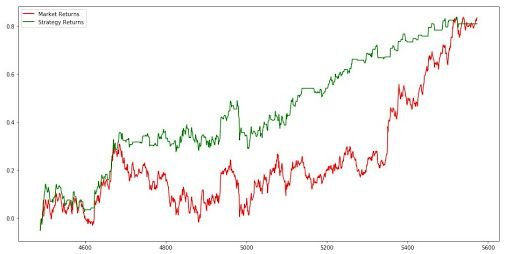## Conclusion

Thus, as we reach the end of the neural network tutorial, we believe that now you can build your own Artificial Neural Network in Python and start trading using the power and intelligence of your machines. Apart from Neural Networks, there are many other machine learning models that can be used for trading. The Artificial Neural Network or any other Deep Learning model will be most effective when you have more than 100,000 data points for training the model.

This model was developed on daily prices to make you understand how to build the model. It is advisable to use the minute or tick data for training the model, which will give you enough data for effective training.

You can enroll for the neural network course on Quantra where you can use advanced neural network techniques and latest research models such as LSTM & RNN to predict markets and find trading opportunities. Keras, the relevant python library is used.